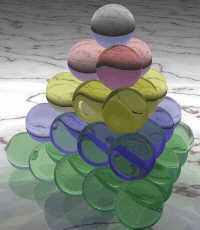# Twelve Days of Christmas and tetrahedral numbers

How many gifts are there in the song Twelve Days of Christmas?

Day 2: 1 + 2 = 3 gifts
Day 3: 1 + 2 + 3 = 6 gifts

Day 12: 1 + 2 + 3 + … + 12 = 78 gifts

The number of gifts on day n is the nth triangular number. The total number of gifts up to and including day n is the sum of the first n triangular numbers, known as the nth tetrahedral number. In the image below, the total number of balls is the fifth tetrahedral number. The number of balls in each layer are triangular numbers. (Image credit: Math is Fun.)I’ll develop a formula for tetrahedral numbers and continuations of the pattern  such as the sum of tetrahedral numbers etc.

First, let T(n, 1) = n.

Next, let T(n, 2) be the nth triangular number. So T(n, 2) is the sum of the first n terms in the sequence T(i, 1).

Next, let T(n, 3) be the nth tetrahedral number. So T(n, 3) is the sum of the first n terms in the sequence T(i, 2).

In general, define T(n, k) to be the sum of the first n terms in the sequence T(i, k-1). You could think of T(n, k) as the nth k-dimensional triangular number. (A tetrahedron is a sort of 3-dimensional triangle. It’s a pyramid whose base is a triangle. T(n,4) would count balls arranged in a sort of 4-dimensional triangle, a simplex in 4 dimensions.)

Theorem: T(n, k) = n(n+1)(n+2) … (n+k-1)/k!

Corollary: There are T(12, 3) = 12*13*14/6 = 364 gifts in the Twelve Days of Christmas.

See these notes for a elementary proof by induction.

Update: Here’s more advanced proof that uses calculus of finite differences.  The more advanced proof requires more background, but it also gives a better idea of how someone might have discovered the formula.

## 9 thoughts on “Twelve Days of Christmas and tetrahedral numbers”

1. Funny that the 12 days of Christmas turn out to have just short of one gift per day for a full year. A coincidence?

As for the tetrahedron being “a sort of 3-dimensional triangle”, if you want to get a little more formal, a tetrahedron is a dimension 3 simplex, just as the triangle is a dimension 2 one. Guess that makes T(n,k) the n-th k dimensional simplicial number…

2. Eric Wilson

Intriguing post. The only weakness is that the theorem seems to come out of nowhere. Here’s the thought process I went through recently.

I was asking myself the same question the other day. My wife was reading a book to our children that “hid” the items from the 12 days of Christmas on each page. The process of finding all of the gifts was tiresome, and drove me to scribbling out a computation before she completed the book.

I didn’t think about tetrahedrons, that’s really helpful. I recognized that each day is a triangular number. Knowing that triangular numbers are a diagonal of Pascal’s triangle, it follows that the tetrahedral numbers, being sums of triangular numbers, would be an adjacent diagonal.

One quickly observes that the desired diagonal is the numbers of the form n choose 3 for some n. Since 3 choose 3 is one it must be that the nth tetrahedral number is n+2 choose 3.

This lead me to conclude that there are 14 choose 3 gifts. I then erroneously computed that to be 1092.

Anyway, the combination of the tetrahedrons and Pascal’s Triangle is fascinating.

Last thought: The number of gifts is also the number of triangles formed placing 14 points on a circle and connecting each point to all of the others. (Not so useful, but there you are.)

3. Eric, I’ve updated the post to link to another proof. The new proof doesn’t “seem to come out of nowhere” as much as the original proof did. The new proof is more advanced but I believe it’s better motivated.

4. You can also derive this by staring at Pascal’s triangle.

1
1 1
1 2 1
1 3 3 1
1 4 6 4 1

If you sum down along any diagonal from (say) left to right, and then take one step down and left, you find the sum. For instance, start at the top and take three steps down-right — 1+1+1 — and then one step down-left to find 3.

Or start at the first “1” on the second row and sum down-right — 1+2+3 — then take one step down-left to find 6.

This works for all diagonals in the triangle, and it is easy to show by induction, given the fact that that every element is the sum of the two directly above it.

But these sums are exactly your k-dimensional triangle numbers. So T(n,k) = (n+k-1)-choose-k = (n+k-1)! / ((n-1)!k!)

P.S. You could have used T(n,0) = 1 for your definition instead of T(n,1) = n :-)

5. Of course, it would look better if my whitespace had not gotten coalesced. Maybe this will look better:

``` 1 1 1 1 2 1 1 3 3 1 1 4 6 4 1 ```

A “preview” function for these comments would be useful.

6. John, great post!

I think there is a small error in the last two formulae of the advanced proof. Should they be like this (http://bit.ly/hzjQCf) or I’m missing something?

7. Let f(n,k) = n(n – 1)…(n – k + 1).

The result follows easily by applying the method of differences to
f(n,k+1) – f(n-1,k+1) = n(n – 1)…(n – k) – (n – 1)(n – 2)…(n – k – 1).
= (k + 1)(n – 1)…(n – k).
= (k + 1) f(n-1,k).

8. Randy A MacDonald

Some APL or J:

+/++n/1

9. Eric Smith

In advanced proof, shouldn’t “for k > 0” be “for k > 1”?